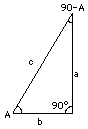Site Map Math Index Glossary Timeline Questions & Answers Lesson Plans #M-9. Deriving sines & cosines

# (M-8) How to tell Sines from Cosines

 Triangles come in many shapes. It would be hard to classify triangles of arbitrary shapes, but we note that any triangle ABC can always be divided into two right angled triangles, triangles with one angle equal to 90°. Those are easier to handle.The three angles inside a triangle add up to 180o (we won't prove it here) and therefore, in a right-angled triangle with sharp angles A and B A + B + 90° = 180° Subtracting 90° from both sides A + B = 90°     Given the value of just one angle A, the other angle B is completely determined (it equals 90°−A), and so is the shape of the triangle, though not its size.     Let the sides of the triangle be named (a,b,c), each matching the name of the angle across from it. The angle A does not determine the length of any side, but it does uniquely fix the ratios between sides. Those ratios have names, and a specific notation exists for writing them: a/c = sin A -- "the sine (or sinus) of A" b/c = cos A -- "the cosine (or cosinus) of A" To tell them apart, just remember: sin A has the side opposite to the angle A on top of its fraction cos A has the side adjacent to the angle A on top of its fraction     A simple connection exists between the sine and cosine of any angle. For by the theorem of Pythagoras a2 + b2 = c2 Therefore, for any angle A (sin A)2 + (cos A)2 = (a2/c2) + (b2/c2) = (a2 + b2)/c2 = 1     This statement is usually written with the square written sin2A (not sinA2, which might be taken to mean the sine of an angle equal to A2): sin2A + cos2A = 1     Both sinA and cosA must be numbers smaller than 1, for the adjacent and opposite sides of a triangle are always shorter than the side across from the 90° angle (called the hypotenuse, a word dear to the lovers of jokes and puns).     As the angle A gets closer and closer to 90° (and B gets smaller and smaller), the triangle becomes increasingly narrow and skinny. The length of side a then approaches that of c, while the length of b becomes very small: therefore as A approaches 90°, sinA approaches 1 and cosA approaches zero. For the derivation of some other values, see the next section.

 By the way: the first table of sines was compiled by Al-Khorezmi, who lived in Baghdad around 780-850 and who also gave us the term algebra. Today's hand-held calculators display their values at the push of a button.     The origin of the name "sine" (in Latin sinus, a bay) is interesting. Like the decimal system, it originally came from India and was adopted by Arab mathematicians around the time of Al-Khorezmi. They transliterated the Indian name for the sine without its vowels (which Arabs did not write) as jb. In 1085 the Castillian (Spanish) king Alphonso the 6th captured Toledo from the Arabs, and with it captured a large library with many Arab manuscripts, including translations of Greek books unknown in the rest of Europe. Alphonso hired scholars who gradually translated those books into Latin.     In 1145 one of those translators, Robert of Chester, translated Al-Khorezmi's "Algebra." At one point in the book he encountered the word "jb" and, not realizing it was a foreign word transliterated into Arabic, looked up what its Arabic meaning might be. With appropriate vowels added, it meant "bay", which in Latin was "sinus." That was what he wrote down and that is the term still used. It is given its proper meaning in medicine (as in "sinus headache") where it means the cavities ("nasal sinuses") extending from the nose towards the eyes.
 (The above story comes from page 96 in a small but delightful book "A History of π (Pi)" by Petr Beckman, St. Martin's Press, 1971.) Other trigonometric functions exist, such as the tangent of A, discussed in section M-12, written tan A = a/b = sinA/cosA Its partner is the "cotangent of A," cotan A = b/a = cosA/sinA The tangent has the opposite side on top, the cotangent the adjacent side.

Author and Curator:   Dr. David P. Stern
Mail to Dr.Stern:   stargaze("at" symbol)phy6.org .

Last updated 25 November 2001